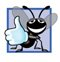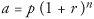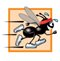# Examples Using the for Statement

The following examples show techniques for varying the control variable in a for statement. In each case, we write the appropriate for header. Note the change in the relational operator for loops that decrement the control variable.

1. Vary the control variable from 1 to 100 in increments of 1.

``` for ( int i = 1; i <= 100; i++ )
```
2. Vary the control variable from 100 to 1 in decrements of 1.

``` for ( int i = 100; i >= 1 ; i-- )
```
3. Vary the control variable from 7 to 77 in increments of 7.

``` for ( int i = 7; i <= 77; i += 7 )
```
4. Vary the control variable from 20 to 2 in decrements of 2.

``` for ( int i = 20; i >= 2; i -= 2 )
```
5. Vary the control variable over the following sequence of values: 2, 5, 8, 11, 14, 17, 20.

``` for ( int i = 2 ; i <= 20; i += 3 )
```
6. Vary the control variable over the following sequence of values: 99, 88, 77, 66, 55, 44, 33, 22, 11, 0.

``` for ( int i = 99; i >= 0; i -= 11 )
```

Common Programming Error 5.6Not using the proper relational operator in the loop-continuation condition of a loop that counts downward (e.g., using i <= 1 instead of i >= 1 in a loop counting down to 1) is usually a logic error.

Application: Summing the Even Integers from 2 to 20

We now consider two sample applications that demonstrate simple uses of for. The application in Fig. 5.5 uses a for statement to sum the even integers from 2 to 20 and store the result in an int variable called total.

Figure 5.5. Summing integers with the for statement.

``` 1 // Fig. 5.5: Sum.java
2 // Summing integers with the for statement.
3
4 public class Sum
5 {
6 public static void main( String args[] )
7 {
8 int total = 0; // initialize total
9
10 // total even integers from 2 through 20
11 for ( int number = 2; number <= 20; number += 2 )
12  total += number;
13
14 System.out.printf( "Sum is %d
", total ); // display results
15 } // end main
16 } // end class Sum
```

 ```Sum is 110 ```

The initialization and increment expressions can be comma-separated lists of expressions that enable the programmer to use multiple initialization expressions or multiple increment expressions. For example, the body of the for statement in lines 1112 of Fig. 5.5 could be merged into the increment portion of the for header by using a comma as follows:

``` for ( int number = 2 ; number <= 20; total += number, number += 2 )
; // empty statement
```

Good Programming Practice 5.4Limit the size of control statement headers to a single line if possible.

Good Programming Practice 5.5Place only expressions involving the control variables in the initialization and increment sections of a for statement. Manipulations of other variables should appear either before the loop (if they execute only once, like initialization statements) or in the body of the loop (if they execute once per iteration of the loop, like increment or decrement statements).

Application: Compound Interest Calculations

The next application uses the for statement to compute compound interest. Consider the following problem:

A person invests \$1,000 in a savings account yielding 5% interest. Assuming that all the interest is left on deposit, calculate and print the amount of money in the account at the end of each year for 10 years. Use the following formula to determine the amounts:where

p is the original amount invested (i.e., the principal)

r is the annual interest rate (e.g., use 0.05 for 5%)

n is the number of years

a is the amount on deposit at the end of the nth year.

This problem involves a loop that performs the indicated calculation for each of the 10 years the money remains on deposit. The solution is the application shown in Fig. 5.6. Lines 810 in method main declare double variables amount, principal and rate, and initialize principal to 1000.0 and rate to 0.05. Java treats floating-point constants like 1000.0 and 0.05 as type double. Similarly, Java treats whole number constants like 7 and -22 as type int.

Figure 5.6. Compound-interest calculations with for.

(This item is displayed on page 189 in the print version)

``` 1 // Fig. 5.6: Interest.java
2 // Compound-interest calculations with for.
3
4 public class Interest
5 {
6 public static void main( String args[] )
7 {
8 double amount; // amount on deposit at end of each year
9 double principal = 1000.0; // initial amount before interest
10 double rate = 0.05; // interest rate
11
13 System.out.printf( "%s%20s
", "Year", "Amount on deposit" );
14
15 // calculate amount on deposit for each of ten years
16 for ( int year = 1; year <= 10; year++ )
17 {
18  // calculate new amount for specified year
19  amount = principal * Math.pow( 1.0 + rate, year );
20
21  // display the year and the amount
22  System.out.printf( "%4d%,20.2f
", year, amount );
23 } // end for
24 } // end main
25 } // end class Interest
```

 ```Year Amount on deposit 1 1,050.00 2 1,102.50 3 1,157.63 4 1,215.51 5 1,276.28 6 1,340.10 7 1,407.10 8 1,477.46 9 1,551.33 10 1,628.89 ```

Line 13 outputs the headers for this application's two columns of output. The first column displays the year, and the second column displays the amount on deposit at the end of that year. Note that we use the format specifier %20s to output the String "Amount on Deposit". The integer 20 between the % and the conversion character s indicates that the value output should be displayed with a field width of 20that is, printf displays the value with at least 20 character positions. If the value to be output is less than 20 character positions wide (17 characters in this example), the value is right justified in the field by default. If the year value to be output were more than four character positions wide, the field width would be extended to the right to accommodate the entire valuethis would push the amount field to the right, upsetting the neat columns of our tabular output. To indicate that values should be output left justified, simply precede the field width with the minus sign () formatting flag.

The for statement (lines 1623) executes its body 10 times, varying control variable year from 1 to 10 in increments of 1. This loop terminates when control variable year becomes 11. (Note that year represents n in the problem statement.)

Classes provide methods that perform common tasks on objects. In fact, most methods must be called on a specific object. For example, to output text in Fig. 5.6, line 13 calls method printf on the System.out object. Many classes also provide methods that perform common tasks and do not require objects. Such methods are called static methods. For example, Java does not include an exponentiation operator, so the designers of Java's Math class defined static method pow for raising a value to a power. You can call a static method by specifying the class name followed by a dot (.) and the method name, as in

ClassName.methodName( arguments )

In Chapter 6, you will learn how to implement static methods in your own classes.

We use static method pow of class Math to perform the compound interest calculation in Fig. 5.6. Math.pow(x, y) calculates the value of x raised to the yth power. The method receives two double arguments and returns a double value. Line 19 performs the calculation a = p (1 + r)n, where a is amount, p is principal, r is rate and n is year.

After each calculation, line 22 outputs the year and the amount on deposit at the end of that year. The year is output in a field width of four characters (as specified by %4d). The amount is output as a floating-point number with the format specifier %,20.2f. The comma (,) formatting flag indicates that the floating-point value should be output with a thousands separator. The actual separator used is specific to the user's locale (i.e., country). For example, in the United States, the number will be output using commas to separate the thousands and a decimal point to separate the fractional part of the number, as in 1,234.45. The number 20 in the format specification indicates that the value should be output right justified in a field width of 20 characters. The .2 specifies the formatted number's precisionin this case, the number is rounded to the nearest hundredth and output with two digits to the right of the decimal point.

We declared variables amount, principal and rateto be of type double in this example. We are dealing with fractional parts of dollars and thus need a type that allows decimal points in its values. Unfortunately, floating-point numbers can cause trouble. Here is a simple explanation of what can go wrong when using double (or float) to represent dollar amounts (assuming that dollar amounts are displayed with two digits to the right of the decimal point): Two double dollar amounts stored in the machine could be 14.234 (which would normally be rounded to 14.23 for display purposes) and 18.673 (which would normally be rounded to 18.67 for display purposes). When these amounts are added, they produce the internal sum 32.907, which would normally be rounded to 32.91 for display purposes. Thus, your output could appear as

``` 14.23
+ 18.67
-------
32.91
```

but a person adding the individual numbers as displayed would expect the sum to be 32.90. You have been warned!

Good Programming Practice 5.6Do not use variables of type double (or float) to perform precise monetary calculations. The imprecision of floating-point numbers can cause errors that will result in incorrect monetary values. In the exercises, we explore the use of integers to perform monetary calculations. [Note: Some third-party vendors provide for-sale class libraries that perform precise monetary calculations. In addition, the Java API provides class java.math.BigDecimal for performing calculations with arbitrary precision floating-point values.]

Note that the body of the for statement contains the calculation 1.0 + rate, which appears as an argument to the Math.pow method. In fact, this calculation produces the same result each time through the loop, so repeating the calculation every iteration of the loop is wasteful.

Performance Tip 5.2In loops, avoid calculations for which the result never changessuch calculations should typically be placed before the loop. [Note: Many of today's sophisticated optimizing compilers will place such calculations outside loops in the compiled code.]Java How to Program (6th Edition) (How to Program (Deitel))
ISBN: 0131483986
EAN: 2147483647
Year: 2003
Pages: 615